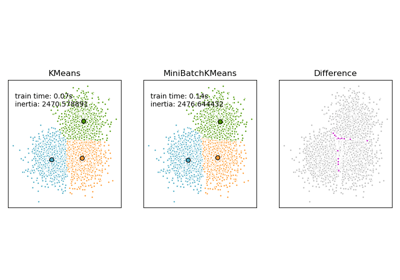/scikit-learn

# sklearn.metrics.pairwise_distances_argmin

`sklearn.metrics.pairwise_distances_argmin(X, Y, axis=1, metric=’euclidean’, batch_size=None, metric_kwargs=None)` [source]

Compute minimum distances between one point and a set of points.

This function computes for each row in X, the index of the row of Y which is closest (according to the specified distance).

This is mostly equivalent to calling:

pairwise_distances(X, Y=Y, metric=metric).argmin(axis=axis)

but uses much less memory, and is faster for large arrays.

This function works with dense 2D arrays only.

Parameters: `X : array-like` Arrays containing points. Respective shapes (n_samples1, n_features) and (n_samples2, n_features) `Y : array-like` Arrays containing points. Respective shapes (n_samples1, n_features) and (n_samples2, n_features) `axis : int, optional, default 1` Axis along which the argmin and distances are to be computed. `metric : string or callable` metric to use for distance computation. Any metric from scikit-learn or scipy.spatial.distance can be used. If metric is a callable function, it is called on each pair of instances (rows) and the resulting value recorded. The callable should take two arrays as input and return one value indicating the distance between them. This works for Scipy’s metrics, but is less efficient than passing the metric name as a string. Distance matrices are not supported. Valid values for metric are: from scikit-learn: [‘cityblock’, ‘cosine’, ‘euclidean’, ‘l1’, ‘l2’, ‘manhattan’] from scipy.spatial.distance: [‘braycurtis’, ‘canberra’, ‘chebyshev’, ‘correlation’, ‘dice’, ‘hamming’, ‘jaccard’, ‘kulsinski’, ‘mahalanobis’, ‘minkowski’, ‘rogerstanimoto’, ‘russellrao’, ‘seuclidean’, ‘sokalmichener’, ‘sokalsneath’, ‘sqeuclidean’, ‘yule’] See the documentation for scipy.spatial.distance for details on these metrics. `batch_size : integer` Deprecated since version 0.20: Deprecated for removal in 0.22. Use sklearn.set_config(working_memory=…) instead. `metric_kwargs : dict` keyword arguments to pass to specified metric function. `argmin : numpy.ndarray` Y[argmin[i], :] is the row in Y that is closest to X[i, :].

## Examples using `sklearn.metrics.pairwise_distances_argmin`Color Quantization using K-MeansComparison of the K-Means and MiniBatchKMeans clustering algorithms